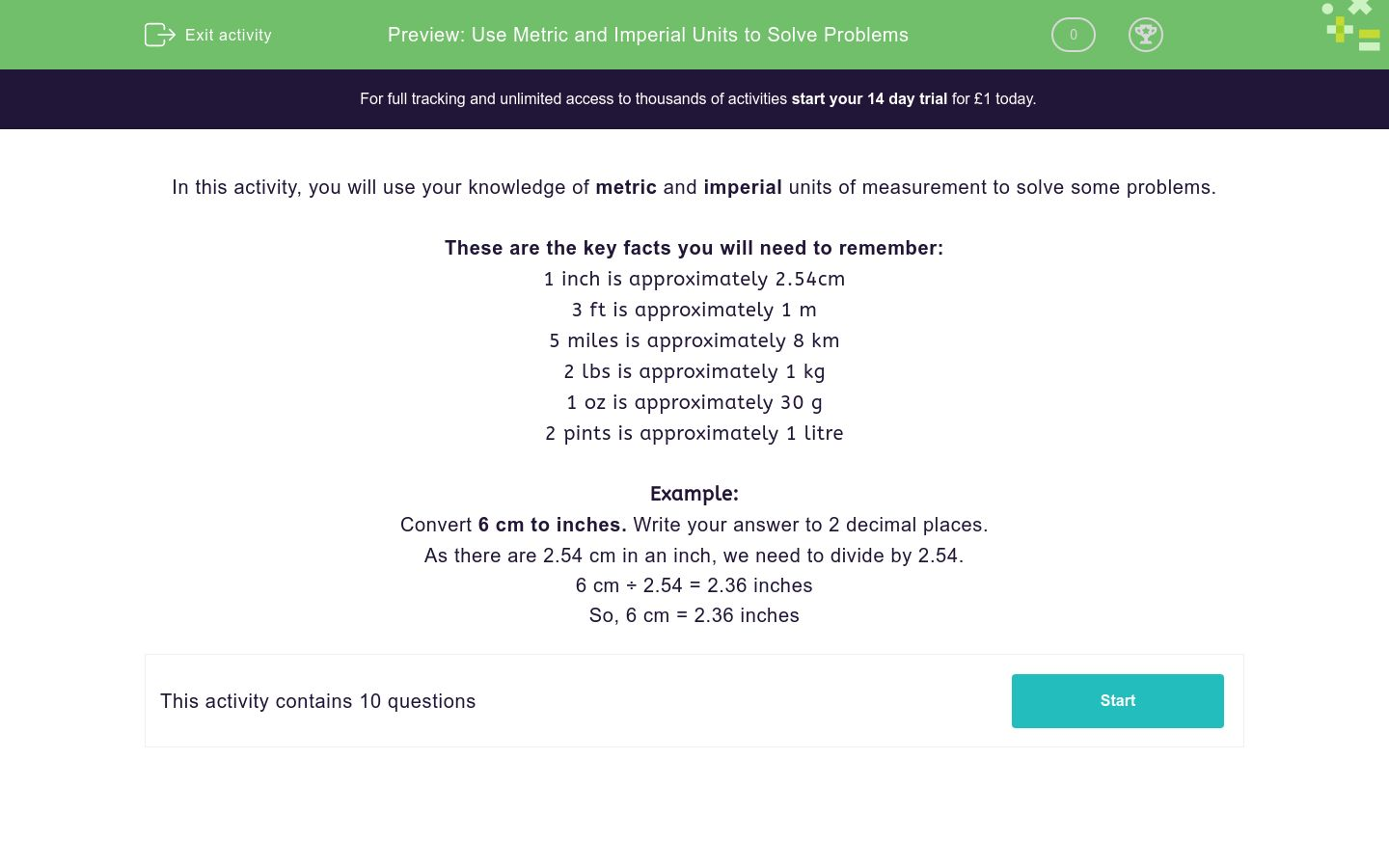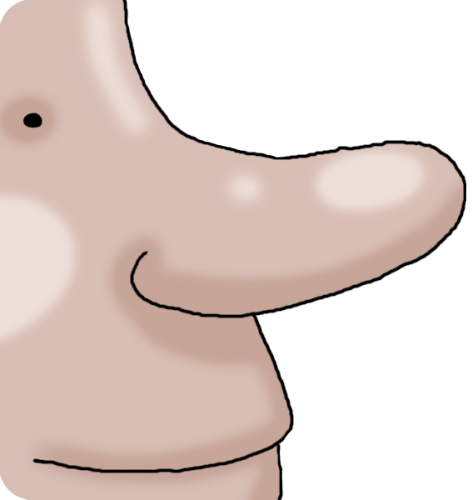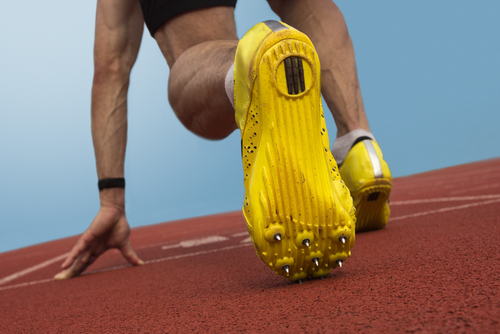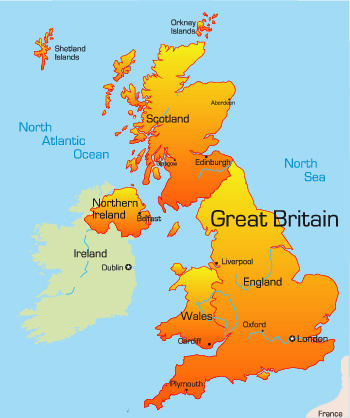# Use Metric and Imperial Units to Solve Problems

In this worksheet, students will be asked to use metric and imperial units. Students will need to be able to convert between the two, in order to solve a range of problems. For some of the questions, the student may require a calculator.Key stage:  KS 2

Curriculum topic:   Measurement

Curriculum subtopic:   Convert Between Metric Measurements

Difficulty level:### QUESTION 1 of 10

In this activity, you will use your knowledge of metric and imperial units of measurement to solve some problems.

These are the key facts you will need to remember:

1 inch is approximately 2.54cm

3 ft is approximately 1 m
5 miles is approximately 8 km
2 lbs is approximately 1 kg
1 oz is approximately 30 g
2 pints is approximately 1 litre

Example:

Convert 6 cm to inches. Write your answer to 2 decimal places.

As there are 2.54 cm in an inch, we need to divide by 2.54.

6 cm ÷ 2.54 = 2.36 inches

So, 6 cm = 2.36 inches

Convert 8 cm to inches.

Remember, 1 inch is approximately 2.54 cm.

3.20 inches

3.15 inches

4.15 inches

6.17 inches

The current world record for the longest nose on a living person is 8.8 cm.

Can you convert this measurement into inches by completing the blank space?

Remember, 1 inch is approximately 2.54 cm3.20 inches

3.15 inches

4.15 inches

6.17 inches

Can you convert 14 inches to cm?

Remember, 1 inch is approximately 2.54cm.

35.56 cm

35.16 cm

36.56 cm

35.05 cm

The largest recorded bubblegum bubble blown has a diameter of 20 inches.

Can you convert this measurement into cm?

Remember, 1 inch is approximately 2.54 cm.35.56 cm

35.16 cm

36.56 cm

35.05 cm

Can you convert 18 feet into metres?

Remember, 3 feet is approximately 1 metre.

 7 Metres 8 Metres 6 Metres 9 Metres 18 Feet = ? Metres

The longest snake in the world is recorded to have measured 25 feet in length.

Remember, 3 feet is approximately 1 metre.

Can you convert this into metres? Give your answer to 2 decimal places.7 Metres 8 Metres 6 Metres 9 Metres 18 Feet = ? Metres

The longest distance ever recorded to have been run in a period of 24 hours is an amazing 350 miles.

What is this distance converted to kilometres?

Remember, 1 mile = approximately 1.6 kilometres.The driving distance from London to Birmingham is approximately 202 kilometres.

Remember, 1 mile is approximately 1.6 km.

Can you convert this distance into miles?126.25 miles

126.14 miles

125.26 miles

127.25 miles

A Spaniel dog weighs 45 lbs.

What does the Spaniel weigh in kg?

Remember, 2 lbs is approximately 1 kg.126.25 miles

126.14 miles

125.26 miles

127.25 miles

A German Shepherd dog weighs 43.2 kg.

What does the German Shepherd weigh in lbs?

Remember, 2 lbs is approximately 1 kg.126.25 miles

126.14 miles

125.26 miles

127.25 miles

• Question 1

Convert 8 cm to inches.

Remember, 1 inch is approximately 2.54 cm.

3.15 inches
EDDIE SAYS
To convert cm to inches, we divide by 2.54 as 1 inch = 2.54 cm. 8cm ÷ 2.54 = 3.15 inches Hopefully, this isn't too difficult now you've read the breakdown in the method. Let's keep practising together.
• Question 2

The current world record for the longest nose on a living person is 8.8 cm.

Can you convert this measurement into inches by completing the blank space?

Remember, 1 inch is approximately 2.54 cmEDDIE SAYS
Again, in order to convert cm to inches, we must divide by 2.54. 8.8 cm ÷ 2.54 = 3.46 inches.
• Question 3

Can you convert 14 inches to cm?

Remember, 1 inch is approximately 2.54cm.

35.56 cm
EDDIE SAYS
As 1 inch = 2.54 cm, we need to multiply by 2.54. 14 x 2.54 = 35.56 cm
• Question 4

The largest recorded bubblegum bubble blown has a diameter of 20 inches.

Can you convert this measurement into cm?

Remember, 1 inch is approximately 2.54 cm.EDDIE SAYS
As 1 inch = 2.54 cm, we need to multiply by 2.54. 20 x 2.54 = 50.8 cm Hopefully, you're getting the hang of this by now.
• Question 5

Can you convert 18 feet into metres?

Remember, 3 feet is approximately 1 metre.

 7 Metres 8 Metres 6 Metres 9 Metres 18 Feet = ? Metres
EDDIE SAYS
To roughly convert 18 feet into metres, we divide by 3. This is because there are approximately 3 feet in 1 metre. 18 feet = approximately 6 metres.
• Question 6

The longest snake in the world is recorded to have measured 25 feet in length.

Remember, 3 feet is approximately 1 metre.

Can you convert this into metres? Give your answer to 2 decimal places.EDDIE SAYS
To roughly convert 25 feet into metres, we divide by 3. This is because there is approximately 3 feet in 1 metre. 25 feet = approximately 8.33 metres.
• Question 7

The longest distance ever recorded to have been run in a period of 24 hours is an amazing 350 miles.

What is this distance converted to kilometres?

Remember, 1 mile = approximately 1.6 kilometres.560 km
560 kilometres
EDDIE SAYS
To roughly convert 350 miles to kilometres, we have to multiply 350 by 1.6 350 x 1.6 = 560. 350 miles = 560 kilometres.
• Question 8

The driving distance from London to Birmingham is approximately 202 kilometres.

Remember, 1 mile is approximately 1.6 km.

Can you convert this distance into miles?126.25 miles
EDDIE SAYS
To convert kilometres to miles, we have to divide by 1.6 as 1 km = 1.6 miles. 202 ÷ 1.6 = 126.25 miles. You're making great progress, two questions to go...
• Question 9

A Spaniel dog weighs 45 lbs.

What does the Spaniel weigh in kg?

Remember, 2 lbs is approximately 1 kg.EDDIE SAYS
We know 2 lbs is approximately 1 kg. Therefore we divide 45 lbs by 2. 45 ÷ 2 = 22.5 kg
• Question 10

A German Shepherd dog weighs 43.2 kg.

What does the German Shepherd weigh in lbs?

Remember, 2 lbs is approximately 1 kg.EDDIE SAYS
We know 2 lbs is approximately 1 kg. Therefore we multiply 43.2 kg by 2. 43.2 x 2 = 86.4 lbs. Great work, you've completed another activity! How about attempting another one so you feel super confident?
---- OR ----

Sign up for a £1 trial so you can track and measure your child's progress on this activity.

### What is EdPlace?

We're your National Curriculum aligned online education content provider helping each child succeed in English, maths and science from year 1 to GCSE. With an EdPlace account you’ll be able to track and measure progress, helping each child achieve their best. We build confidence and attainment by personalising each child’s learning at a level that suits them.

Get started